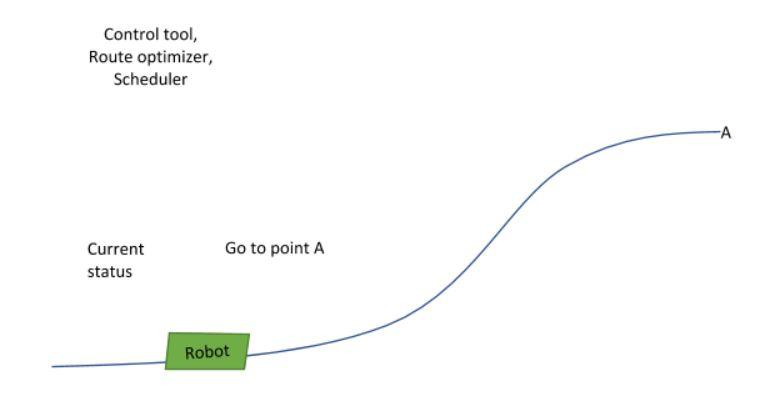# Study of robot route optimization using genetic algorithm

Master of Science thesis • Author: Viljami Männikkö • Read more

My Master of Science thesis “Spline optimization: Spline optimization using a genetic algorithm in AGV system”, written at Atostek, examines spline optimization from the point of view of robot vehicle route optimization. Because the thesis was made for the mathematics department of Tampere University, it focuses on the mathematical basis for the optimization. The objective was to see if genetic algorithms are suitable for finding the optimal shape for the spline when the system has multiple different restrictions.

### Introduction: Robot vehicles and predefined routes

The use of robots and robot vehicles is increasing in the industrial sector. Automatic guided vehicles (AGVs) can be considered to be one kind of robot vehicles because they can operate independently without a driver. These vehicles are needed, for example, at automatic warehouses where they handle routine transportation of goods. We want to make sure that handling of goods is as efficient as possible. Therefore vehicle routes should be optimal. AGV navigation can be handled in multiple ways, such as laser triangulation or floor wiring. Common for all navigation methods is that routes require mapping.

One way to define routes is to use splines, which are polynomial curves. A route network can be constructed by combining multiple splines between pickup and delivery points. An AGV follows the predefined routes by taking commands from its operating system. This study focuses on optimizing those predefined routes.

In all cases of complex route networks with multiple restrictions, finding an optimal route by hand is difficult, whereas using an algorithm for optimizing the AGV route between two given points makes the process quick and efficient.Fig 1. Robot controlling system, where the robot drives a predefined route by taking commands from its control system.

Using splines to model routes has several advantages. Splines are easy to create and handle as they are constructed by using a recursion formula. Also, their shape is easy to modify because it is defined by using control points. Other properties that make it possible for splines to be used to describe vehicle routes are discussed in more detail in my thesis.

### Finding the optimal route for a robot by using a genetic algorithm

A genetic algorithm is a heuristic algorithm that mimics the process of natural selection in nature. In this study, this algorithm was applied to the optimization process. This algorithm has four main phases, which are: Initialization phase, Final phase, Selection phase, and Reproduction phase.

In the Initialization phase, the starting population is created by using the first “candidate”. In this context this means that we generate multiple routes between the starting and ending points. For the route generation, we use the original route of the robot and only modify its control points.

The second phase is the so-called Final phase. This phase name is a bit misleading. In this phase, all algorithm end criteria are checked and if one of the end criteria is met, the algorithm stops. This phase must always be executed after a population evaluation in order to stop the algorithm as soon as the optimal solution is found and before a new population is generated.

The third phase is the Selection phase. In this phase, every candidate is evaluated and then the best candidates from the population are selected as the basis for a new population. In this context this means that the speed graph of each route is evaluated, each drive time is calculated and all collisions to obstacles are detected. After the evaluation, the population’s routes are sorted according to their evaluated fitness value. To determine the fitness value, the drive time of routes plus the penalties for their restriction violations is used.

The fourth phase is the Reproduction phase, which contains a new population creation process. In this process, new candidates are created by using the candidates of the previous population. We create new routes by taking random routes from the previous population and combining them to form new routes. The figure 2 illustrates the optimization process of the algorithm.Fig 2. Genetic algorithm process in current robot route optimization context.

The phases 2-4 are repeated until one of the end criteria is met. After one of the end criteria is met, the best candidate of the last population is the optimal solution. Genetic algorithms are best suited for solving combinatorial problems, but they can also be applied to other kinds of mathematical problems such as this.

### Results of the study

In this study, we created an algorithm able to optimize the robot route according to drive time. In many cases, the algorithm found a much better route for the robot than the original route was. The conclusion is that the genetic algorithm used was well-suited for this problem and for optimizing the spline.

There are a few weaknesses in using a genetic algorithm for solving this kind of problems. The failings are discussed in more detail in the thesis. Because of them, other approaches to the solving of this optimization problem are also introduced in the thesis.

The developed algorithm has multiple other applications, as well. For example, the algorithm can be applied to finding the fastest route between two given points. This study gives a basis for all kinds of spline optimization. By changing the algorithm so that it takes new restrictions into account it can be applied to many other problems. The only precondition is that the optimized property can be defined by a spline.Viljami Männikkö
Software designer